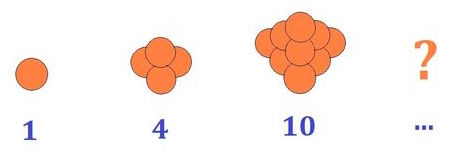# Can you count all these oranges?

Probability Level 2The oranges are arranged such that there is 1 top orange; the second top layer has 2 more oranges than the top; the third has 3 more oranges than the second, and so on. Forming a tetrahedron of oranges, these "tetrahedral" numbers of oranges run as a series, as shown above.

What is the value of the $100^{\text{th}}$ term of this series?

×